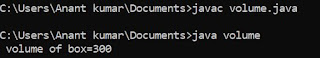## Sunday, 1 April 2018

### Program in C to add,sub,multi,div.of two number.

This is the program in C to input two numbers and calculate all mathematical operation .Here firstly we have to enter two number from the user i.e a and b and then operation should be done .For that firstly we have declare three variable at the starting of the program and then input the number .Now we are going to start the writing the program.

#include<stdio.h>
void main()
{
int a,b,c;
clrscr();
printf ("\n Enter the value of a:");
scanf ("%d",& a);
printf ("\n Enter the value of b:");
scanf ("%d",& b);
c= a + b;
printf ("\n sum =%d",c);
c= a - b;
printf ("\n sub =%d",c);
c = a * b;
printf ("\n multi =%d",c);
c = a / b;
printf ("\n div =%d",c);
getch();
}